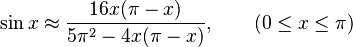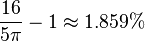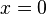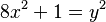# Bhāskara I facts for kids

Kids Encyclopedia Facts
Quick facts for kids
Bhāskara I
Born c. 600 CE
Died c. 680 CE
Nationality Indian
Occupation Mathematician; scientist
Known for Bhaskara I's sine approximation formula

Bhāskara (c. 600 – c. 680) (commonly called Bhaskara I to avoid confusion with the 12th-century mathematician Bhāskara II) was a 7th-century mathematician and astronomer, who was the first to write numbers in the Hindu decimal system with a circle for the zero, and who gave a unique and remarkable rational approximation of the sine function in his commentary on Aryabhata's work. This commentary, Āryabhaṭīyabhāṣya, written in 629 CE, is among the oldest known prose works in Sanskrit on mathematics and astronomy. He also wrote two astronomical works in the line of Aryabhata's school, the Mahābhāskarīya and the Laghubhāskarīya.

On 7 June 1979 the Indian Space Research Organisation launched Bhaskara I honouring the mathematician.

## Biography

Little is known about Bhāskara's life. He was probably an astronomer. He was born in India in the 7th century.There are references to places in India in Bhaskara's writings. For example he mentions Valabhi (today Vala), the capital of the Maitraka dynasty in the 7th century, and Sivarajapura, which were both in Saurastra which today is the Gujarat state of India on the west coast of the continent. Also mentioned are Bharuch (or Broach) in southern Gujarat and Thanesar in the eastern Punjab which was ruled by Harsa for 41 years from 606. Harsa was the pre-eminent ruler in north India through the first half of Bhaskara I's life. A reasonable guess would be that Bhaskara was born in Saurastra and later moved to Asmaka.

His astronomical education was given by his father. Bhaskara is considered the most important scholar of Aryabhata's astronomical school. He and Brahmagupta are two of the most renowned Indian mathematicians who made considerable contributions to the study of fractions.

## Representation of numbers

Bhaskara's probably most important mathematical contribution concerns the representation of numbers in a positional system. The first positional representations had been known to Indian astronomers approximately 500 years prior to this work. However, these numbers, prior to Bhaskara, were written not in figures but in words or allegories and were organized in verses. For instance, the number 1 was given as moon, since it exists only once; the number 2 was represented by wings, twins, or eyes since they always occur in pairs; the number 5 was given by the (5) senses. Similar to our current decimal system, these words were aligned such that each number assigns the factor of the power of ten correspondings to its position, only in reverse order: the higher powers were right from the lower ones.

His system is truly positional since the same words representing, can also be used to represent the values 40 or 400. Quite remarkably, he often explains a number given in this system, using the formula ankair api ("in figures this reads"), by repeating it written with the first nine Brahmi numerals, using a small circle for the zero . Contrary to his word system, however, the figures are written in descending values from left to right, exactly as we do it today. Therefore, at least since 629, the decimal system is definitely known to the Indian scientists. Presumably, Bhaskara did not invent it, but he was the first having no compunctions to use the Brahmi numerals in a scientific contribution in Sanskrit.

## Mathematics

Bhaskara wrote three astronomical contributions. In 629 he annotated the Aryabhatiya, written in verses, about mathematical astronomy. The comments referred exactly to the 33 verses dealing with mathematics. There he considered variable equations and trigonometric formulae.

His work Mahabhaskariya divides into eight chapters about mathematical astronomy. In chapter 7, he gives a remarkable approximation formula for sin x, that is$\sin x \approx \frac{16x (\pi - x)}{5 \pi^2 - 4x (\pi - x)}, \qquad (0 \leq x \leq \pi )$

which he assigns to Aryabhata. It reveals a relative error of less than 1.9% (the greatest deviation$\frac{16}{5\pi} - 1 \approx 1.859\%$ at$x=0$). Moreover, relations between sine and cosine, as well as between the sine of an angle >90° >180° or >270° to the sine of an angle <90° are given. Parts of Mahabhaskariya were later translated into Arabic.

Bhaskara already dealt with the assertion that if p is a prime number, then 1 + (p–1)! is divisible by p. It was proved later by Al-Haitham, also mentioned by Fibonacci, and is now known as Wilson's theorem.

Moreover, Bhaskara stated theorems about the solutions of today so-called Pell equations. For instance, he posed the problem: "Tell me, O mathematician, what is that square which multiplied by 8 becomes - together with unity - a square?" In modern notation, he asked for the solutions of the Pell equation$8x^2 + 1 = y^2$. It has the simple solution x = 1, y = 3, or shortly (x,y) = (1,3), from which further solutions can be constructed, e.g., (x,y) = (6,17).

## Astronomy

The Mahabhaskariya comprises of eight chapters dealing with mathematical astronomy. The book deals with topics such as: the longitudes of the planets; association of the planets with each other and also with the bright stars; the lunar crescent; solar and lunar eclipses; and rising and setting of the planets.Bhāskara I Facts for Kids. Kiddle Encyclopedia.